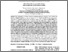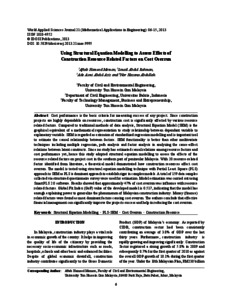# Using Structural Equation Modelling to Assess Effects of Construction Resource Related Factors on Cost Overrun

Memon, Aftab Hameed and Rahman, Ismail Abdul and Aziz, Ade Asmi Abdul and Abdullah, Nor Hazana (2013) Using Structural Equation Modelling to Assess Effects of Construction Resource Related Factors on Cost Overrun. World Applied Sciences Journal, Vol.21 (01). pp. 6-15. ISSN 18184952Preview
Text (pdf)
TSI-Artikel-005 Using Structural Equation Modelling to Assess Effects.pdf - Published Version

Download (322kB) | Preview
Official URL: http://www.idosi.org

## Abstract

ost performance is the basic criteria for measuring success of any project. Since construction projects are highly dependable on resources, construction cost is significantly affected by various resource related factors. Compared to traditional methods of data analysis, Structural Equation Model (SEM) is the graphical equivalent of a mathematical representation to study relationship between dependant variable to explanatory variable. SEM is regarded as extension of standardized regression modelling and is important tool to estimate the causal relationship between factors. SEM functionality is better than other multivariate techniques including multiple regression, path analysis and factor analysis in analyzing the cause-effect relations between latent constructs. Since no study has estimated causal relations among resource factors and cost performance yet, hence this study adopted structural equation modelling to assess the effects of the resource related factors on project cost in the southern part of peninsular Malaysia. With 20 resource-related factor identified from literature, a theoretical model demonstrated how construction resources affect cost overrun. The model is tested using structural equation modelling technique with Partial Least Square (PLS) approach to SEM as PLS is dominant approach to establish rigor in complex models. A total of 159 data samples collected via structured questionnaire survey were used for estimation. Model estimation was carried out using SmartPLS 2.0 software. Results showed that approximately 47% of cost overrun was influence with resource related factors. Global Fit Index (GoF) value of the developed model is 0.517, indicating that the model has enough explaining power to generalize the phenomenon of Malaysian construction industry. Money (finance) related factors were found as most dominant factors causing cost overrun. The authors conclude that effective financial management can significantly improve the projects success and help in reducing the cost overrun

Item Type: Article Construction Resource; Cost Overrun; PLS-SEM; Structural Equation Modelling Civil Engineering > Construction MaterialCivil Engineering > Structural Analysis Fakultas Teknik dan Ilmu Komputer > Program Studi Teknik Sipil Ahmad Yani 25 Jul 2016 02:42 25 Jul 2016 02:42 http://repository.bakrie.ac.id/id/eprint/134

### Actions (login required)View Item